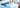Practice Numerical Reasoning TestHow to Calculate Compound Interest

Updated September 27, 2021

What Is Compound Interest?

Compound interest is an important concept to understand, as it will help you appreciate how your savings (or debts) might accrue over time.

Here’s an explanation that may help:

You put £1,000 of savings into a bank account that pays 5% annually.

How much do you have after a year? That’s an easy one: £1,050.

But what about at the end of year two?

The answer is not £1,100, because your interest has compounded: in other words, it has been calculated on the combination of principal plus interest.

Over the second year you have earned 5% interest on £1,050. So you now have £1,102.50.

After 10 years? £1,628.89.

After twenty years? £2,653.30. You’ve almost tripled your money, and it’s because of the power of compound interest.

Golden rule: the longer the investment time, the more compound interest will generate significant returns (or significant losses, if we’re talking about unpaid debt).When Might I Need to Calculate Compound Interest?

There are many instances apart from calculating returns on savings or repayments on loans.

Perhaps you have applied for a job in the financial services sector, or maybe you are sitting an aptitude test for a job.

Understanding compound interest can also allow you to work out if it makes sense to accept discounts from suppliers on early payments.

Formulas for Calculating Compound Interest

A typical formula for compounding interest will look something like this:

A = P (1 + RN)nt

Don’t be put off! Let’s break down what that means.

In this equation, the letters relate to:

• A – The future value of the investment or loan, which also includes interest
• P – Principal investment amount; for example, the initial deposit or amount of a loan
• R – Interest rate as a decimal
• N – The number of times in the year that the interest is compounded
• T – The time in years (usually relating to the length of the investment or loan)

Say, for example, that £5,000 was placed into a savings account which accrued interest of 5% that was compounded monthly.

We’d calculate the value of the investment after a 10-year period as follows:

P = £5,000

R = 0.5

N would, therefore, be 12 and T would be 10

So putting these figures into the formula:

A = 5,000 (1 + 0.05/12) to the power of (12(10)) = £8,235.05

The first formula will provide you with the future amount of a loan or investment that includes the compound interest in addition to the principal.

If you wish to calculate the compound interest figure only, you can use this formula:

Compounded Interest = P (1+½)nt – P

As explained previously, the idea behind compound interest is that the interest is added back to the primary figure, so interest is earned on the collective amount before the interest is then added in the next compounding period.

Two Sample Questions

If you’re having to answer questions on compound interest as part of an aptitude test, make sure you really know how to use the two formulas given above.

Typically you’ll be asked to calculate the level of interest, the total amount or the period of investment.

Sample Question 1

The compound interest on £30,000 at 7% per year is £4,347. How long was the investment?

a) 2 years
b) 2.5 years
c) 3 years

To reach the answer you would work through the formula in the following way:

A = £30,000 + £4,347 = £34,347

The time in years is T

So using the formula A = P (1 + RN )nt:

£34,347 = £30,000 (1 + 7/1)nt

£34,347/£30,000 = (1 + 7/1)nt

£1.1449 = (1 + 7/1)nt

£1.1449*(1 + 7/1) = nt

As we know n = 1, £1.1449 8 1.7 = 2

Therefore, the time of the investment is 2 years.

Sample Question 2

If £3,500 is invested at 10% each year and compounded each month, how much money will be in the account if the investment is held for 8 years?

a) £7,776.25
b) £7,763.37
c) £7,812.15

The first step is to put the figures into the equation:

A = P (1 + r/n) nt

So we have:

• P, or the amount invested, as £3,500
• R is the interest rate at 10%
• N is the number of times the interest is compounded annually, which is 12
• T is the time of the investment, which is 8 years

Using the above equation this would translate to:

A = £3,500 (1 + 0.1/12) 12*8

A= £3,500 * 2.218105

A= £7,763.37

Common Mistakes and Areas to Look out For

Calculating compound interest can prove a challenge, even for the most numerate. One common mistake, for example, is to work out the amount rather than the interest rate, or vice versa.

If you’re calculating compound in a test, you need to become astute at reading the question accurately. It is always worth re-reading it to make sure that you have understood.

Don’t be reticent to make use of paper for rough notes. For compound interest questions this is even more important, since there are usually several components to work out. Writing things down can also help reduce the likelihood of an error creeping into your calculations.

For the majority of numerical tests, one scenario applies to multiple questions that follow. As such, it’s worth investing time to really understand that scenario (and the data presented) before you answer each question.